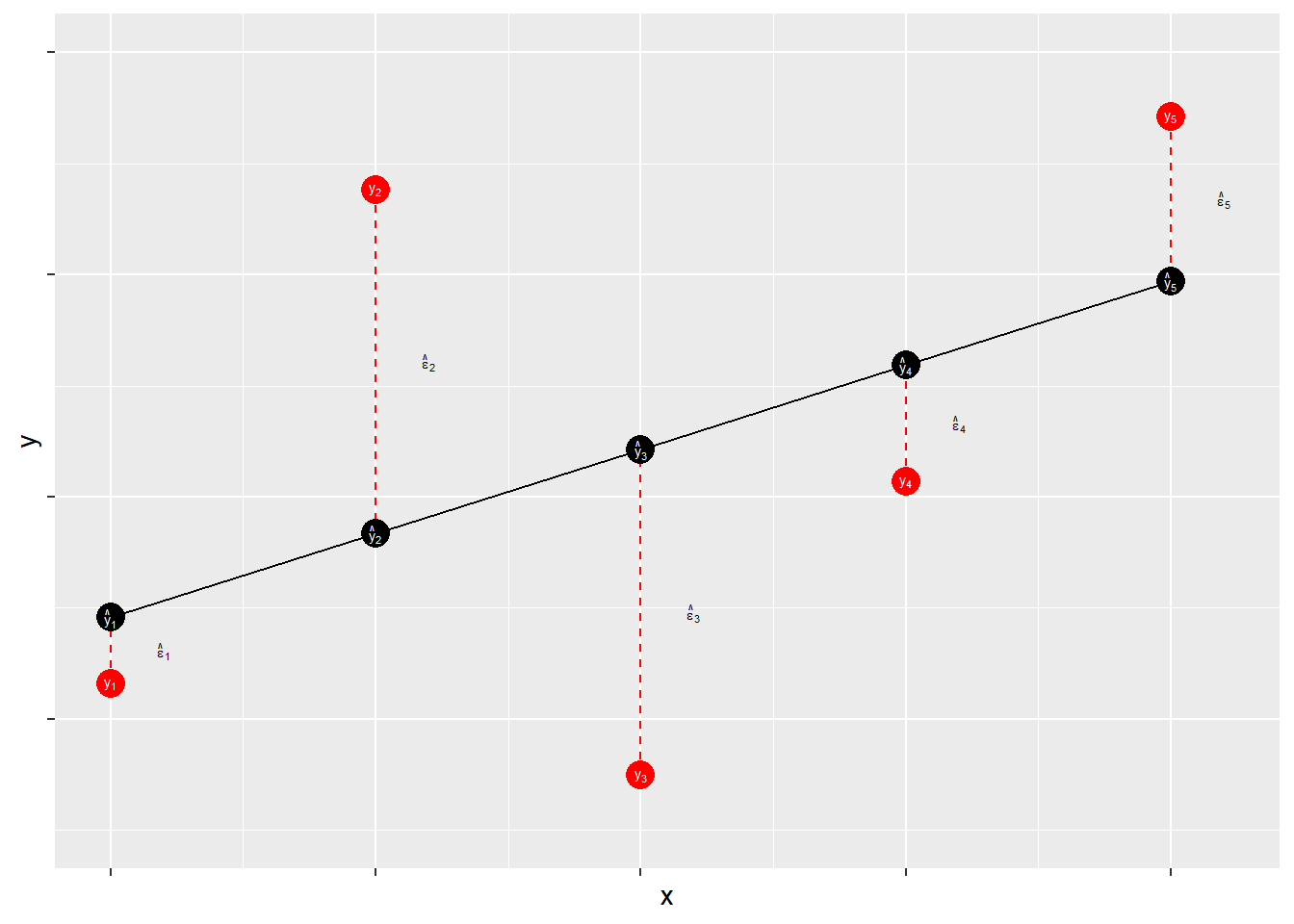# 7.2: Estimating Linear Models

With stochastic models we don’t know if the error assumptions are met, nor do we know the values of αα and ββ; therefore we must estimate them, as denoted by a hat (e.g., ^αα^ is the estimate for αα). The stochastic model as shown in Equation (7.4) is estimated as:

Yi=^α+^βXi+ϵi(7.4)(7.4)Yi=α^+β^Xi+ϵi

where ϵiϵi is the residual term or the estimated error term. Since no line can perfectly pass through all the data points, we introduce a residual, ϵϵ, into the regression equation. Note that the predicted value of YY is denoted ^YY^ (yy-hat).

Yi=^α+^βXi+ϵi=^Yi+ϵiϵi=Yi−^Yi=Yi−^α−^βXiYi=α^+β^Xi+ϵi=Yi^+ϵiϵi=Yi−Yi^=Yi−α^−β^Xi

## 7.2.1 Residuals

Residuals measure prediction errors of how far observation YiYi is from predicted ^YiYi^. This is shown in Figure $$\PageIndex{3}$$.Figure $$\PageIndex{3}$$: Residuals: Statistical Forensics

The residual term contains the accumulation (sum) of errors that can result from measurement issues, modeling problems, and irreducible randomness. Ideally, the residual term contains lots of small and independent influences that result in an overall random quality of the distribution of the errors. When that distribution is not random – that is, when the distribution of error has some systematic quality – the estimates of ^αα^ and ^ββ^ may be biased. Thus, when we evaluate our models we will focus on the shape of the distribution of our errors.

What’s in ϵϵ?

Measurement Error

• Imperfect operationalizations
• Imperfect measure application

Modeling Error

• Modeling error/mis-specification
• Missing model explanation